# Area of a piecewise rectangular figure Online Quiz

#### Complete Python Prime Pack

9 Courses     2 eBooks

#### Artificial Intelligence & Machine Learning Prime Pack

6 Courses     1 eBooks

#### Java Prime Pack

9 Courses     2 eBooks

Following quiz provides Multiple Choice Questions (MCQs) related to Area of a piecewise rectangular figure. You will have to read all the given answers and click over the correct answer. If you are not sure about the answer then you can check the answer using Show Answer button. You can use Next Quiz button to check new set of questions in the quiz.Q 1 - Find the area of piecewise rectangular figure given below.### Explanation

Step 1:

Area of a rectangle = l × w; l = length; w = width

Step 2:

Area of 2 piecewise rectangles = 4 × 3 + 7 × 4 = 40 square units

Q 2 - Find the area of piecewise rectangular figure given below.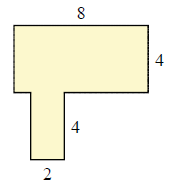### Explanation

Step 1:

Area of a rectangle = l × w; l = length; w = width

Step 2:

Area of 2 piecewise rectangles = 4 × 2 + 8 × 4 = 40 square units

Q 3 - Find the area of piecewise rectangular figure given below.### Explanation

Step 1:

Area of a rectangle = l × w; l = length; w = width

Step 2:

Area of 2 piecewise rectangles = 2 × 3 + 6 × 5 = 36 square units

Q 4 - Find the area of piecewise rectangular figure given below.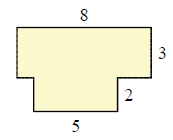### Explanation

Step 1:

Area of a rectangle = l × w; l = length; w = width

Step 2:

Area of 2 piecewise rectangles = 8 × 3 + 5 × 2 = 34 square units

Q 5 - Find the area of piecewise rectangular figure given below.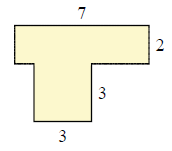### Explanation

Step 1:

Area of a rectangle = l × w; l = length; w = width

Step 2:

Area of 2 piecewise rectangles = 3 × 3 + 7 × 2 = 23 square units

Q 6 - Find the area of piecewise rectangular figure given below.### Explanation

Step 1:

Area of a rectangle = l × w; l = length; w = width

Step 2:

Area of 2 piecewise rectangles = 4 × 5 + 5 × 2 = 30 square units

Q 7 - Find the area of piecewise rectangular figure given below.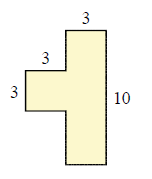### Explanation

Step 1:

Area of a rectangle = l × w; l = length; w = width

Step 2:

Area of 2 piecewise rectangles = 3 × 3 + 3 × 10 = 39 square units

Q 8 - Find the area of piecewise rectangular figure given below.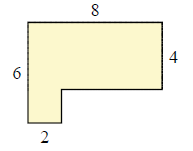### Explanation

Step 1:

Area of a rectangle = l × w; l = length; w = width

Step 2:

Area of 2 piecewise rectangles = 4 × 8 + 2 × 2 = 36 square units

Q 9 - Find the area of piecewise rectangular figure given below.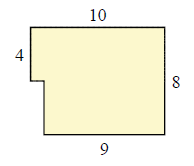### Explanation

Step 1:

Area of a rectangle = l × w; l = length; w = width

Step 2:

Area of 2 piecewise rectangles = 4 × 9 + 10 × 4 = 76 square units

Q 10 - Find the area of piecewise rectangular figure given below.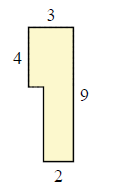### Explanation

Step 1:

Area of a rectangle = l × w; l = length; w = width

Step 2:

Area of 2 piecewise rectangles = 4 × 3 + 5 × 2 = 22 square units

area_of_piecewise_rectangular_figure.htm